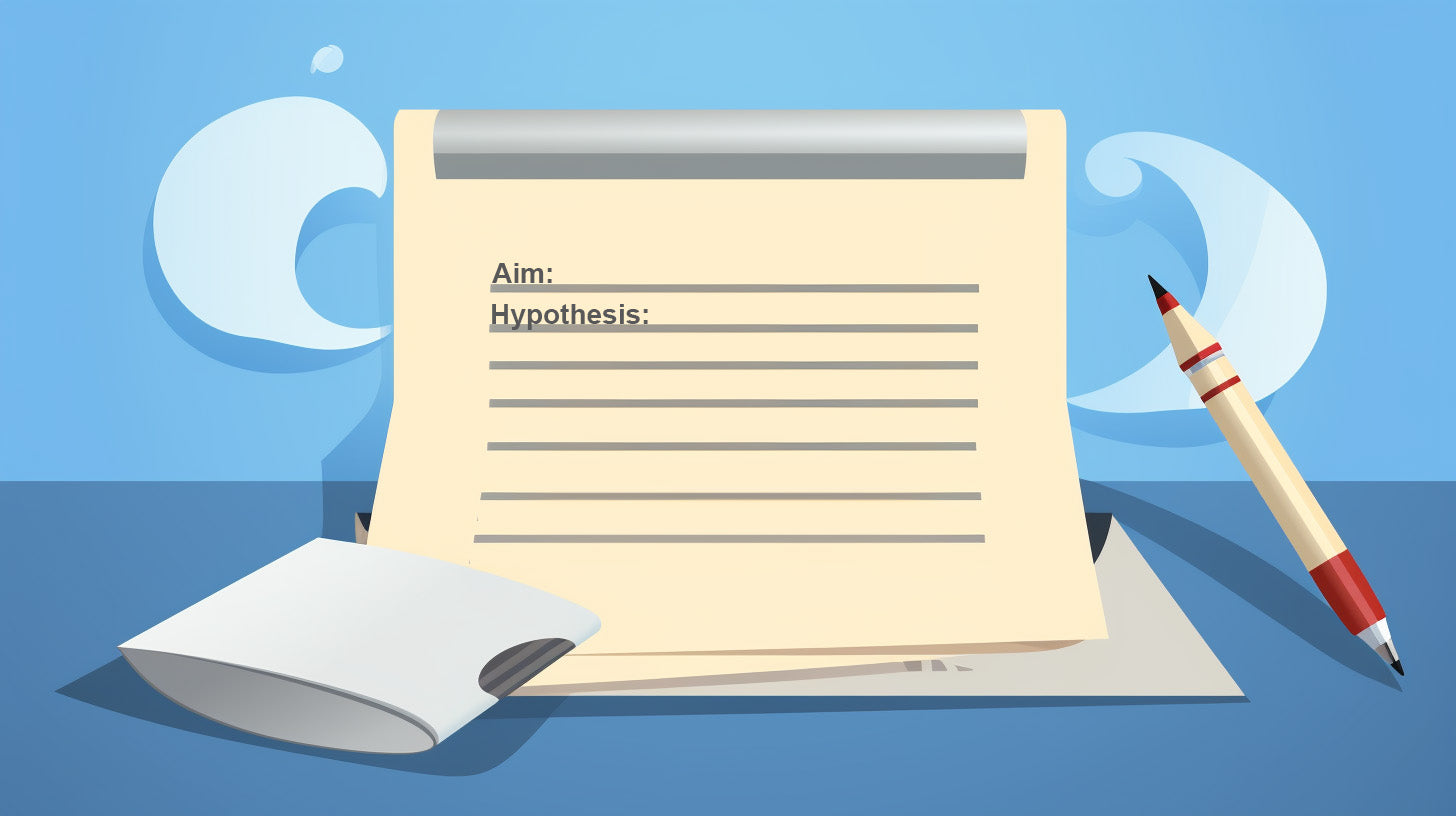# How to Prepare for Practical Exams

Practical examinations are an integral part of HSC Chemistry and Physics curricula. They demand a different approach compared to theoretical exams as they are designed to test your understanding of concepts in real-world scenarios. Preparation for these exams requires a clear understanding of the experimental procedures, theories behind those experiments, the aim and hypothesis, graph interpretations, and more. In this blog post, we will explore effective strategies to ace your practical exams.

###Start your preparation by revisiting the experiments related to the concepts that will be examined. Generally, the syllabus dot points with the verb 'investigate' means there is a practical element you must know. Familiarise yourself with the procedural steps, equipment used, and the safety precautions.

With regards to looking at the experiments from the examined module consider the following:

### Understand the Theory Behind the Experiments

Every experiment is grounded in fundamental principles and theories. For instance, in Chemistry, the titration experiment is based on the principle of neutralisation reaction. Therefore, make sure you understand these underlying theories as they provide the rationale for the steps followed in the experiments.

### What are the Practical Steps and What is Their Importance

Knowing the procedural steps of an experiment is not enough. You should also understand why each step is carried out and its significance in the context of the experiment. This understanding helps you make correct decisions during the experiment, especially when things don't go as planned.

### Aim and Hypothesis of ExperimentThe aim outlines what the experiment intends to accomplish or discover, while the hypothesis is your predicted outcome based on your understanding of the theory.

Understanding these aspects is crucial, as some practical exams may require you to write your own aim and hypothesis. Alternatively, you could also be asked to design an experiment for a given aim and hypothesis.

### Validity, Accuracy, and Reliability of Experiments

Understanding what validity, accuracy, and reliability are, as well as how to assess each one, is essential. This is as they are almost always asked in practical exams.

Validity refers to whether the experiment measures what it is intended to measure. Accuracy refers to the closeness of the experimental result to the true or accepted value, while reliability is about the consistency of results in repeated trials.

You are very likely to be asked to assess the validity, accuracy or reliability of experimental results or ways to improve each one. As such, it is a good idea to brainstorm ideas prior to the exam. Ask yourself questions such as:

What are some possible errors in the experimental design that could decrease accuracy?

How could I change the experimental design to address these errors?

Are the experimental results likely to be replicable? If not, what part of the experimental design could be causing the inconsistencies?

###Graphs are powerful tools that can illustrate trends, patterns, and relationships in data. It's crucial to understand what kind of graph can be drawn for each experiment (line or curve of best fit), the variables plotted, and how to interpret them.

On that note, consider the following:

The gradient or slope of a graph can yield meaningful information in scientific experiments. In Physics, the slope of a distance-time graph gives speed, while in Chemistry, the gradient of a Beer-Lambert plot provides the absorption coefficient. Understanding how to use the gradient is key to interpreting your experimental results.

Once you have understood what the gradient of a particular graph represents, you should seek examples where you can calculate the gradient. In physics, you may be asked to calculate Planck's constant from a kinetic energy vs frequency graph. In chemistry, you may be asked to calculate the reaction rate of a reaction from a concentration vs time graph.

### 'Visualise' the Practical Aspects

Lastly, while practicing theory questions, try to visualise the practical aspects. Rather than remembering the details of practicals with words and sentences, try to recall the steps from when you actually performed it in class. You may find it more helpful to memorise the details using visuals than texts.

For example, if a question is about the effect of temperature on reaction rate, imagine the setup of the corresponding experiment, like a heated flask with reactants changing colour over time.

This practice will help you make connections between theory and practice, enhancing your overall understanding and performance in the exam.

To conclude, practical exams in HSC Chemistry and Physics require a well-rounded understanding of the experiments, not just rote memorisation. By applying the strategies outlined above, you can confidently approach your practical exams and demonstrate your scientific proficiency. Happy studying!